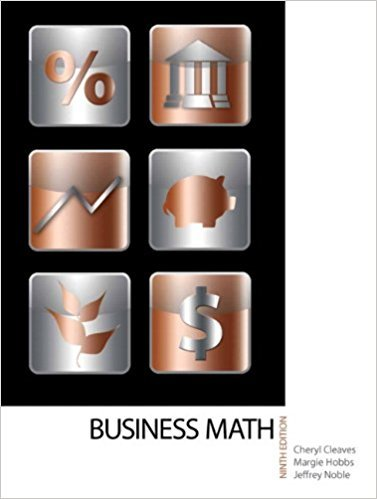×
×

# Multiply. 73.7 * 0.02ISBN: 9780135108178 355

## Solution for problem 13 Chapter 3-2

• Textbook Solutions
• 2901 Step-by-step solutions solved by professors and subject experts
• Get 24/7 help from StudySoup virtual teaching assistants4 5 1 383 Reviews
17
0
Problem 13

Multiply. 73.7 * 0.02

Step-by-Step Solution:
Step 1 of 3

L26 - 2 Consider the graph of f(x)s kcedbel: Where is the function f(x)c ocveupanddown Test for Concavity ▯▯ Assume...

Step 2 of 3

Step 3 of 3

##### ISBN: 9780135108178

Unlock Textbook Solution